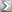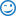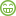# Interesting Math Problemby Kurt
32 replies
• |
Here's an interesting math problem being shared on social media...

1 + 4 = 5
2 + 5 = 12
3 + 6 = 21
5 + 8 = ?

The issue I have with this is that I believe there's at least 3 possible correct answers for this...What do you think?
• 5+8 = 13 Incorrect answers to other 'problems' don't change that.

The idea is for someone to become interested in what they think is a bigger pattern and add the number '21' to the answer of '13'?

So then you would have 3 wrong answers rather than 2?
Signature
Saving one dog will not change the world - but the world changes forever for that one dog
***
One secret to happiness is to let every situation be
what it is instead of what you think it should be.
{{ DiscussionBoard.errors.message }}
• Banned

(What you're doing is adding the +Numbers to the previous Result Number.) So 2 + 5 [added to 5] = 12.
Signature
"Each problem has hidden in it an opportunity so powerful that it literally dwarfs the problem. The greatest success stories were created by people who recognized a problem and turned it into an opportunity."―Joseph Sugarman
{{ DiscussionBoard.errors.message }}
•  Originally Posted by Jonathan 2.0The answer is 34. (What you're doing is adding the +Numbers to the previous Result Number.) So 2 + 5 [added to 5] = 12.
That's what I got. But I don't see the other possible answers. Although I completely understand why Kay posted what she did.

 Originally Posted by Kay King5+8 = 13 Incorrect answers to other 'problems' don't change that. The idea is for someone to become interested in what they think is a bigger pattern and add the number '21' to the answer of '13'? So then you would have 3 wrong answers rather than 2?
That's such a stunningly obvious answer that I might be convinced that it's right. I was following the pattern rather than the obvious answer. I wonder if it's a male/female thing?
Signature
One Call Closing book https://www.amazon.com/One-Call-Clos...=1527788418&sr

What if they're not stars? What if they are holes poked in the top of a container so we can breath?
{{ DiscussionBoard.errors.message }}
•  Originally Posted by KurtHere's an interesting math problem being shared on social media... 1 + 4 = 5 2 + 5 = 12 3 + 6 = 21 5 + 8 = ? The issue I have with this is that I believe there's at least 3 possible correct answers for this...What do you think?
What are the other two possible answers...besides 34?
Signature
One Call Closing book https://www.amazon.com/One-Call-Clos...=1527788418&sr

What if they're not stars? What if they are holes poked in the top of a container so we can breath?
{{ DiscussionBoard.errors.message }}
•  Originally Posted by Claude WhitacreWhat are the other two possible answers...besides 34?
Kay gave one: 13
J 2.0 gave another: 34

Another is 43. Take the first number, multiply it with the second, then add the first. This formula works for each of the first three:
(x*y) + x = n

There may be other possibilities I haven't thought of...
Signature
Discover the fastest and easiest ways to create your own valuable products.
Tons of FREE Public Domain content you can use to make your own content, PLR, digital and POD products.
{{ DiscussionBoard.errors.message }}
•  Originally Posted by KurtKay gave one: 13 J 2.0 gave another: 34 Another is 43. Take the first number, multiply it with the second, then add the first. This formula works for each of the first three: (x*y) + x = n There may be other possibilities I haven't thought of...
That formula would make it 45 (5 x 8 + 5)

And if we are to accept that the numbers are in a logical sequence, then the answer using J 2.0's calculation should also be 45. That's because if the first three equations are in a sequence, the fourth in that sequence has to be 4 + 7 = 32. The sequence just jumps one place.

If there isn't a missing fourth line, then 5 + 8 must be randomly chosen, and justifies Kay's original answer.
Signature

{{ DiscussionBoard.errors.message }}
• Yes, that formula works...but that is not the formula used in the OP example.

The OP example was a straightforward set of additions - there were not further formulaic instructions.

I'm no math wiz - never was - but I do know the more common way to make a mistake with numbers is to assume something not stated in the formula. I know that because I did it...a lot.

1 + 4 = 5
2 + 5 = 7
3 + 6 = 9
5 + 8 = 13

(1+4)+(2+5)+(3+6)+(5+8) = 34
Signature
Saving one dog will not change the world - but the world changes forever for that one dog
***
One secret to happiness is to let every situation be
what it is instead of what you think it should be.
{{ DiscussionBoard.errors.message }}
• Banned
Thanks Kay. I see what Kurt means now. There are several solutions.

: )
Signature
"Each problem has hidden in it an opportunity so powerful that it literally dwarfs the problem. The greatest success stories were created by people who recognized a problem and turned it into an opportunity."―Joseph Sugarman
{{ DiscussionBoard.errors.message }}
•  Originally Posted by Kay KingYes, that formula works...but that is not the formula used in the OP example. The OP example was a straightforward set of additions - there were not further formulaic instructions. I'm no math wiz - never was - but I do know the more common way to make a mistake with numbers is to assume something not stated in the formula. I know that because I did it...a lot. 1 + 4 = 5 2 + 5 = 7 3 + 6 = 9 5 + 8 = 13 (1+4)+(2+5)+(3+6)+(5+8) = 34

I tend to agree that 13 is the "most correct" answer. But of course it's not the formula given, as that could be what the exercise is all about, finding the formula from the given info to determine the correct answer. I say not enough info was given, therefore it's best to determine all possibilities and that it's possible that all the equations given are correct if we adjust the formatting a bit of each:

1 +4 = 5
2 +5 = 12
3 +6 = 21
5 +8 = ?
Signature
Discover the fastest and easiest ways to create your own valuable products.
Tons of FREE Public Domain content you can use to make your own content, PLR, digital and POD products.
{{ DiscussionBoard.errors.message }}
• I came up with 37 as an answer.

1 + (4 x 1) = 5
2 + (5 x 2) = 12
3 + (6 x 3) = 21
5 + (8 x 4) = 37 ***
{{ DiscussionBoard.errors.message }}
•  Originally Posted by wyatt2011I came up with 37 as an answer. 1 + (4 x 1) = 5 2 + (5 x 2) = 12 3 + (6 x 3) = 21 5 + (8 x 4) = 37 ***
Inventive. I never saw that coming.
Signature
One Call Closing book https://www.amazon.com/One-Call-Clos...=1527788418&sr

What if they're not stars? What if they are holes poked in the top of a container so we can breath?
{{ DiscussionBoard.errors.message }}
• Why 8x4? Shouldn't it be 8x5?
{{ DiscussionBoard.errors.message }}
• Kurt -

I don't necessarily agree with that. You can only do math based on the actual problem presented. Without instructions indicating there is more to the answer - the answer is 13.

A simple line often included (on intelligence tests) with questions like this is "from the numbers below, extrapolate the most logical answer"....then you would have 34.

In this case - maybe that is the tricky part? Perhaps the point is to lead people to add their own interpretation to what is a straight forward problem....and arrive at a wrong answer.
Signature
Saving one dog will not change the world - but the world changes forever for that one dog
***
One secret to happiness is to let every situation be
what it is instead of what you think it should be.
{{ DiscussionBoard.errors.message }}
• Banned
 Originally Posted by Kay KingI don't necessarily agree with that. You can only do math based on the actual problem presented. Without instructions indicating there is more to the answer - the answer is 13.
Hmm. I disagree with that Kay. There's absolutely nothing to suggest that your way of "solving" the formula is the correct one. (It's just your interpretation of the answer based on the presentation.)

For instance most people would interpret 1+1=2. However that's just their conditioned response. Depending on the variable it could also mean 1+1=11.
Signature
"Each problem has hidden in it an opportunity so powerful that it literally dwarfs the problem. The greatest success stories were created by people who recognized a problem and turned it into an opportunity."―Joseph Sugarman
{{ DiscussionBoard.errors.message }}
•  Originally Posted by Kay KingKurt - I don't necessarily agree with that. You can only do math based on the actual problem presented. Without instructions indicating there is more to the answer - the answer is 13. A simple line often included (on intelligence tests) with questions like this is "from the numbers below, extrapolate the most logical answer"....then you would have 34. In this case - maybe that is the tricky part? Perhaps the point is to lead people to add their own interpretation to what is a straight forward problem....and arrive at a wrong answer.
You don't necessarily agree with me when I said "I tend to agree" with you? Interesting.I strongly disagree with your comment:
 "from the numbers below, extrapolate the most logical answer"....then you would have 34.
In this case there's no reason to assume 34 is any more correct than 43, since they both fit.
Signature
Discover the fastest and easiest ways to create your own valuable products.
Tons of FREE Public Domain content you can use to make your own content, PLR, digital and POD products.
{{ DiscussionBoard.errors.message }}
•  Originally Posted by KurtYou don't necessarily agree with me when I said "I tend to agree" with you? Interesting.It's not that you are wrong. It's that you are disagreeable.

And while I'm on a roll.... (joke given freely)

I'm also almost positive that you are still a virgin. And for that, all women are grateful.
Signature
One Call Closing book https://www.amazon.com/One-Call-Clos...=1527788418&sr

What if they're not stars? What if they are holes poked in the top of a container so we can breath?
{{ DiscussionBoard.errors.message }}
• Kurt - This is Sunday. I try not to agree with anyone on Sunday. Have no idea why that is...

OK - just realized it's still Saturday - been a loooong day - so I agree with you.
Signature
Saving one dog will not change the world - but the world changes forever for that one dog
***
One secret to happiness is to let every situation be
what it is instead of what you think it should be.
{{ DiscussionBoard.errors.message }}
• I see 2 possible answers to the conundrum:

1) Half past nine;
2) Quantum physics.
Signature
Why do garden gnomes smell so bad?
So that blind people can hate them as well.
{{ DiscussionBoard.errors.message }}
• Applying the pattern to the last equation gives the answer of 96.

Jason
{{ DiscussionBoard.errors.message }}
• the difference between the last answer and next answer increases 2 each time
5*2+2=12
6*3+3=21=9
7*4+4=32=11
8*5+5=45=13
9*6+6=60=15
10*7+7=77=17
11*8+8=96=19

79*76+76=6080
80*77+77=6237 =157
81*78+78=6396=159

so just say
1*4+1=5
2*6=12
3*7=21
4*8=32
5*9=45
6*10=60
7*11=77
8*12=96

76*80=6080
77*81=6237
78*82=6396

i wonder if mr miller is proud of me finally
{{ DiscussionBoard.errors.message }}
•  Originally Posted by hardraysnightthe difference between the last answer and next answer increases 2 each time 5*2+2=12 6*3+3=21=9 7*4+4=32=11 8*5+5=45=13 9*6+6=60=15 10*7+7=77=17 11*8+8=96=19 79*76+76=6080 80*77+77=6237 =157 81*78+78=6396=159 so just say 1*4+1=5 2*6=12 3*7=21 4*8=32 5*9=45 6*10=60 7*11=77 8*12=96 76*80=6080 77*81=6237 78*82=6396 i wonder if mr miller is proud of me finally{{ DiscussionBoard.errors.message }}
• I would say 45.
Signature

Cheers, Laurence.

{{ DiscussionBoard.errors.message }}
• 1 + 4*1 = 5
2 + 5*2 = 12
3 + 6*3 = 21
5 + 8*5 = 45
Signature

Creative Designer and Developer !

{{ DiscussionBoard.errors.message }}
• Interesting for who?Signature
{{ DiscussionBoard.errors.message }}
• I always end up with 34 lol
{{ DiscussionBoard.errors.message }}
• Banned
 Originally Posted by Elizabeth BoujardI always end up with 34 lol
(Lol) Maybe the answer to the ultimate question of Life (and The Universe) is 42.

(Hehe)
Jonathan
Signature
"Each problem has hidden in it an opportunity so powerful that it literally dwarfs the problem. The greatest success stories were created by people who recognized a problem and turned it into an opportunity."―Joseph Sugarman
{{ DiscussionBoard.errors.message }}
• 1 ? 4 = 5
2 ? 5 = 12
3 ? 6 = 21
4 ? 7 = 32
5 ? 8 = 45
{{ DiscussionBoard.errors.message }}
• Since this is presented as a math problem I am with Kay. The answer is 13.

If the OP presented this as a secret code for messages between two spies I might agree with some of the other answers. Except there is not enough data. Any good spy knows you should always present enough data so the intended reader can only come up with ONE interpretation to your message.
{{ DiscussionBoard.errors.message }}
• Banned
 Originally Posted by Janice SperrySince this is presented as a math problem I am with Kay. The answer is 13. If the OP presented this as a secret code for messages between two spies I might agree with some of the other answers. Except there is not enough data. Any good spy knows you should always present enough data so the intended reader can only come up with ONE interpretation to your message.
I think the beauty of the equation is that it can be interpreted in several different ways. (And, perhaps, why it's been so popular on Social Media.)
Signature
"Each problem has hidden in it an opportunity so powerful that it literally dwarfs the problem. The greatest success stories were created by people who recognized a problem and turned it into an opportunity."―Joseph Sugarman
{{ DiscussionBoard.errors.message }}
• None of the above. The correct answer is 41. Observe:

1 + 4 = 5, which expressed in base 6 is also 5.
2 + 5 = 7, which expressed in base 5 is 12 (1 five and 2 ones).
3 + 6 = 9, which expressed in base 4 is 21 (2 fours and 1 one).
5 + 8 = 13, which expressed in base 3 is 41 (4 threes and 1 one).

Why yes, I did drive my math teachers crazy. Why do you ask?
Signature
Put MY voice on YOUR video: AwesomeAmericanAudio.com
{{ DiscussionBoard.errors.message }}
• 5+8=31

Calculated according to the above rules
{{ DiscussionBoard.errors.message }}
• 5 + 8 = 34
{{ DiscussionBoard.errors.message }}

### Trending Topics

Hey fellow content creators! I've been working hard on my YouTube channel and am eager to grow my subscriber count. I'm on a tight budget, so I'm looking for effective ... [read more]

• ### Domain, DNS Problem

Ali Gürtuna

I bought my domain from godaddy.com years ago. I have not changed my domain register company for years. However, I have changed the company I buy hosting service from many ... [read more]

• ### How to move a prospect through the sales funnel?

BluesPlayer

Hi all, it seems to me that the name of the game in ecommerce marketing is being able to successfully move the prospect through the sales funnel. The key is ... [read more]

• ### Not able to publish in Google News

Ali Gürtuna

Hi, My WordPress site was being published in Google News, albeit not very frequently, until 3 months ago. However, after making some changes to the settings of the Rank Math ... [read more]

• ### I can't remove the deleted pages of my WordPress website from Google Search Console

Ali Gürtuna

Hi, I can't remove the deleted pages of my WordPress website from Google Search Console. Under titles like Blocked by robots.txt Redirect page Not found (404) Excluded by "noindex" tag ... [read more]